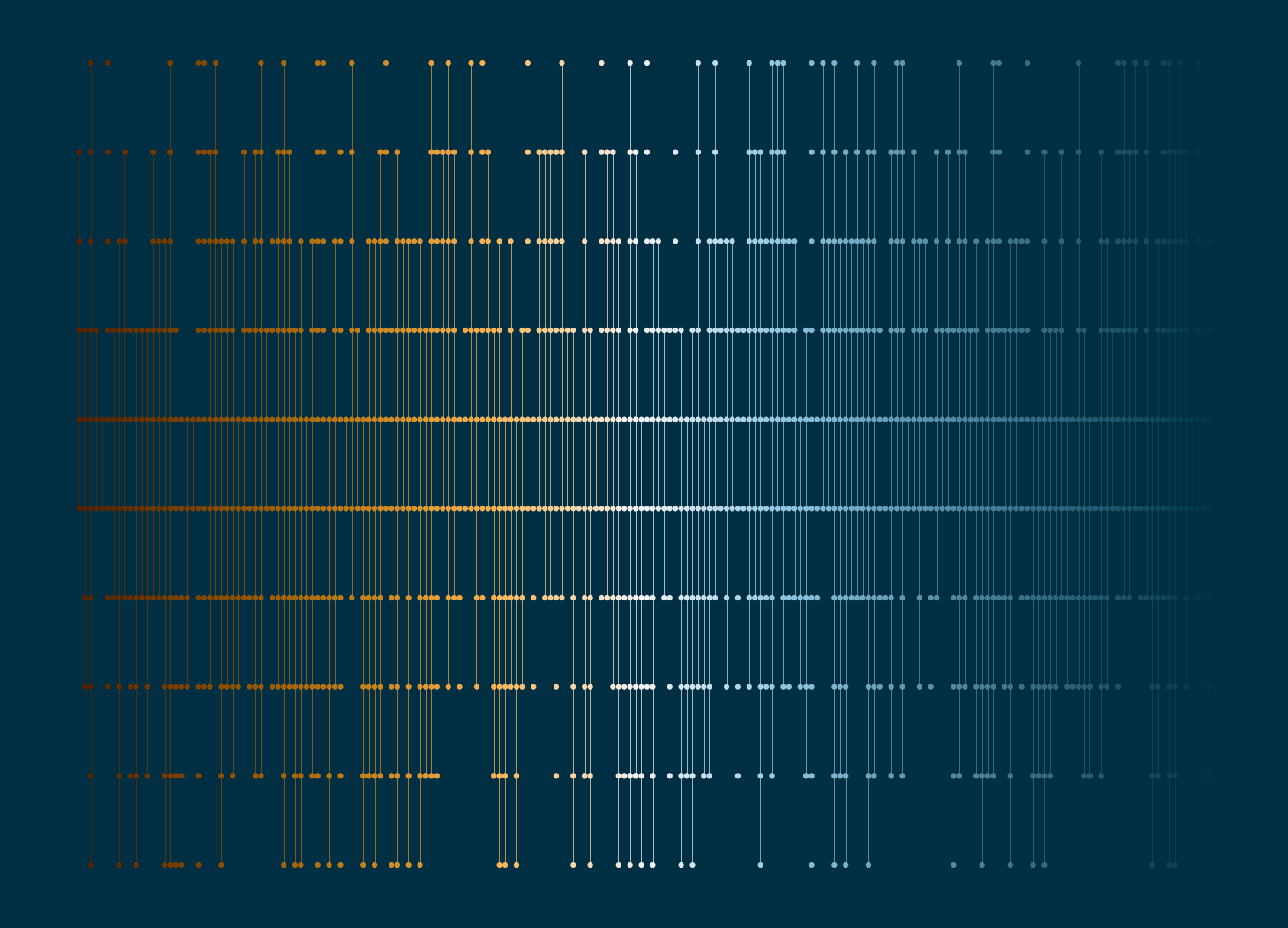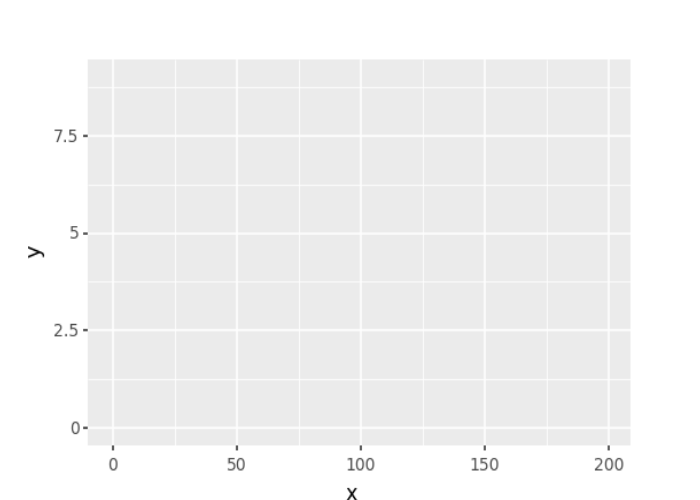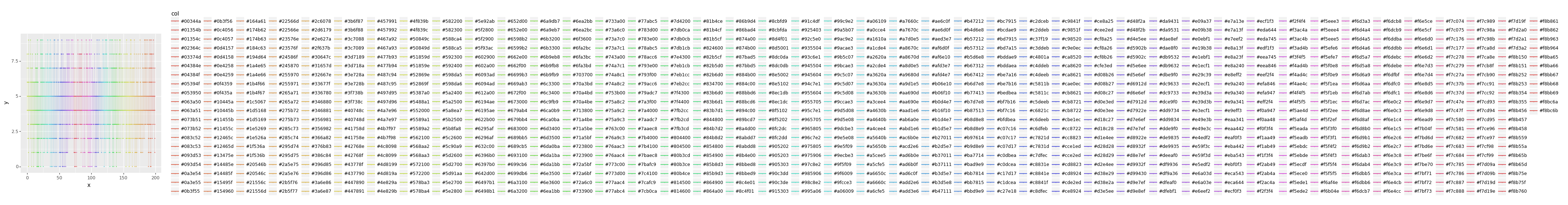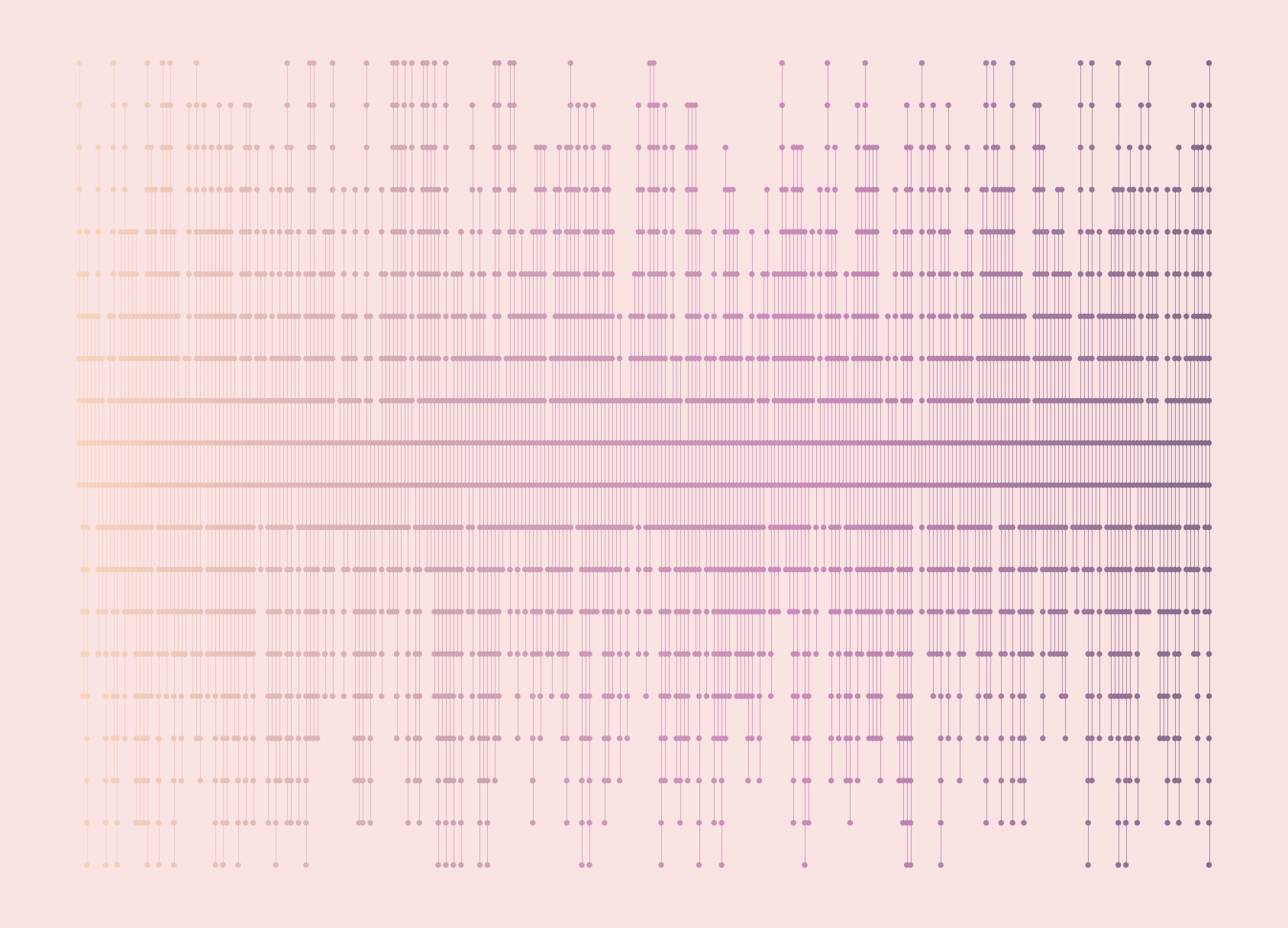Generative art is the process of creating artwork through a set of pre-determined rules often with an element of randomness - art with algorithms. And Python is great for implementing algorithms, so we can use it to make art! We’re going to walk through step-by-step how to make the following piece of art in Python:Let’s start by importing the packages we’ll need:

 ``````1 2 3 4 `````` ``````import random import pandas as pd import matplotlib.colors as mcolors import plotnine as pn ``````

We’ll use `random` for generating random samples of data, `pandas` for manipulating our generated data, `matplotlib.colors` for generating random colours, and `plotnine` for visualising.

I’ve seen `import plotnine as p9` or `import plotnine as gg` used as alternatives to `pn` and there doesn’t seem to be a strong consensus on which one to use, so use whichever you like!

We’re later going to create a function that generates this piece of art, so let’s save ourselves some time by setting up some parameters that we’ll later use as function arguments:

 ``````1 2 3 4 5 6 7 `````` ``````n_x=200 max_y=10 size=0.001 linewidth=0.1 bg_col="#002e42" col_palette=["#552000", "#8a4d00", "#c17d17", "#f8b150", "#f5f5f5", "#93c6e1", "#5f93ac", "#2e627a", "#00344a"] s=1234 ``````

Here, `n_x` is the number of vertical lines; `max_y` is the maximum height of the vertical lines; `size` is the size of the points; `linewidth` is the width of the vertical lines; `bg_col` is the background colour; `col_palette` is a list of hex colours that we’ll use to colour the lines and points; and `s` is the random seed to make sure we can reproduce our art twice!

### The mathematics of art

When I first started making generative art, I quickly realised there was a lot more maths involved than I initially thought. Generally we have a combination of rules and randomness. In this art piece these take the following forms:

Randomness:

• the start point of each vertical line
• the end point of each vertical line

Rules:

• lines start and end within some range (between 0 and some upper value)
• the line should start in the first half of the range, and end in the second half
• points should be drawn equally spaced between each the start and end points of each line

Let’s start with the random elements! We start by setting a `random.seed()` to make sure we can always recreate our art. The start points are always integer values between 0 and half of our `max_y` value. The end points are similar but exist in the upper half of the range. We use `range` to generate a sequence of possible start/end points, and then the `random.choices()` function to sample from that sequence and set the start and end points for each of our `n_x` vertical lines.

 ``````1 2 3 4 `````` ``````# start and end points random.seed(s) n_y_start=random.choices(range(round(max_y/2)), k=n_x) n_y_end=random.choices(range(round(max_y/2)+1, max_y + 1), k=n_x) ``````

Now we can move onto the rules

Here, we want to use the start and end points to construct the x and y coordinates where the plotted points will go (and where the lines will connect). For each vertical line, the y-cordinates will be an integer sequence between the start and end values. The x-coordinate will be a vector of the same length, with a repeated value denoting the number of the vertical line.

 ``````1 2 3 4 5 `````` ``````x_list=[] y_list=[] for i in range(n_x): x_list.extend([i]*len(range(n_y_start[i], n_y_end[i]))) y_list.extend(range(n_y_start[i], n_y_end[i])) ``````

We can then create a `pandas` dataframe to prepare our data for plotting:

 ``````1 `````` ``````plot_data = pd.DataFrame({'x': x_list, 'y': y_list}) ``````

Finally, we need to decide what colours to use! We have our list of hex colours, and what we can do is interpolate between those colours to get a smooth gradient using `mcolors.LinearSegmentedColormap`. The `mcolors.to_hex()` function then converts them to hex values that we can pass into our plotting functions later on.

 ``````1 2 3 `````` ``````# choose colours cmap=mcolors.LinearSegmentedColormap.from_list('custom_cmap', col_palette, N=len(plot_data.index)) plot_data['col']=[mcolors.to_hex(cmap(i)) for i in range(len(plot_data.index))] ``````

### Visualisation with plotnine

The `plotnine` library is a Python implementation of a grammar of graphics based on the {ggplot2} R package. It allows you to create plots by mapping variables in a dataframe to the visual aspects that make up the plot.

Let’s create an initial plot by using the `pn.ggplot()` function. We need to specify two arguments: (i) which data set we want to plot in the `data` argument; and (ii) which columns in the data should be mapped to each plot element in the `mapping` argument. Here, we (unsurprisingly) put the `x` values on the x-axis, and the `y` values on the y-axis. Since we want to draw only vertical lines, rather than connect all points, we also using the `group` argument and group by the `x` values to draw one line for each unique `x` value. We also set map the colour column `col` to the colour element. Note that this all happens inside the `pn.aes()` function - `aes` being short for aesthetics.

 ``````1 `````` ``````(pn.ggplot(data=plot_data, mapping=pn.aes(x="x", y="y", group="x", colour="col"))) ``````This gives us very little so far. We have a plotting grid with the axes limits and labels, but no actual points or lines yet. We can add geometries i.e. plotting shapes using different `geom_` functions. We add points with `pn.geom_point()` and lines with `pn.geom_line()`. We can control the size of the points and the width of the points using the `size` argument inside each of these functions.

 ``````1 2 3 `````` ``````(pn.ggplot(data=plot_data, mapping=pn.aes(x="x", y="y", group="x", colour="col")) + pn.geom_line(size=linewidth) + pn.geom_point(size=size)) ``````There’s one very clear issue with this plot - there’s a huge legend on the right hand side! Also, the colours aren’t actually the ones we wanted to use. These two problems are actually related and can be solved with one additional line: add `pn.scale_colour_identity()` to set the colour scale equal to the values in the dataframe. Since the colour elements are no longer mapped to data values, the legend will disappear as well.

The grey background and axis lines also aren’t very useful in the context of art, and so we want to remove them completely using `pn.theme_void()`. Instead, we can add a different colour to the background with a custom theme. The `plot_background` argument of `pn.theme()` allows us to add our own background colour.

 ``````1 2 3 4 5 6 `````` ``````(pn.ggplot(data=plot_data, mapping=pn.aes(x="x", y="y", group="x", colour="col")) + pn.geom_line(size=linewidth) + pn.geom_point(size=size) + pn.scale_colour_identity() + pn.theme_void() + pn.theme(plot_background=pn.element_rect(fill=bg_col, colour=bg_col))) ``````### Building a function

We can create multiple different versions of our art by varying the different parameters. It’s much easier to do this if we create a function first:

 `````` 1 2 3 4 5 6 7 8 9 10 11 12 13 14 15 16 17 18 19 20 21 22 23 24 25 26 `````` ``````def art(n_x, max_y, size, linewidth, bg_col, col_palette, s): """Generates plot of lines and points.""" # generate data random.seed(s) # start and end points random.seed(s) n_y_start=random.choices(range(round(max_y/2)), k=n_x) n_y_end=random.choices(range(round(max_y/2)+1, max_y + 1), k=n_x) # get x and y co-ordinates in dataframe x_list=[] y_list=[] for i in range(n_x): x_list.extend([i]*len(range(n_y_start[i], n_y_end[i]))) y_list.extend(range(n_y_start[i], n_y_end[i])) plot_data = pd.DataFrame({'x': x_list, 'y': y_list}) # choose colours cmap=mcolors.LinearSegmentedColormap.from_list('custom_cmap', col_palette, N=len(plot_data.index)) plot_data['col']=[mcolors.to_hex(cmap(i)) for i in range(len(plot_data.index))] # plot data p = (pn.ggplot(data=plot_data, mapping=pn.aes(x="x", y="y", group="x", colour="col")) + pn.geom_line(size=linewidth) + pn.geom_point(size=size) + pn.scale_colour_identity() + pn.theme_void() + pn.theme(plot_background=pn.element_rect(fill=bg_col, colour=bg_col))) return p ``````

Then we can finally call our function:

 ``````1 `````` ``````p = art(n_x=200, max_y=10, size=0.001, linewidth=0.1, bg_col="#002e42", col_palette=["#552000", "#8a4d00", "#c17d17", "#f8b150", "#f5f5f5", "#93c6e1", "#5f93ac", "#2e627a", "#00344a"], s=1234) ``````and save a copy as a PNG with `pn.ggsave()`:

 ``````1 `````` ``````pn.ggsave(p, filename="Images/nexus.png", height=5, width=7, dpi=300) ``````

or try different values for the arguments!

 ``````1 `````` ``````art(n_x=300, max_y=20, size=0.001, linewidth=0.1, bg_col="#FAE3E3", col_palette=["#F7D4BC", "#CFA5B4", "#C98BB9", "#846B8A"], s=1234) ``````### Other resources

• The `plotnine` documentation provides instructions for creating plots using `plotnine` and there are plenty of examples in the Gallery section.

• I previously wrote a blog post about Best (artistic) practices in R, and pretty much everything written there also applies to art in Python.

• Geoffrey Bradway wrote a blog post about plotter art using voronoi diagrams that uses `matplotlib` instead of `plotnine` for visualisation.Image: giphy.com